### Coloring Formulas

The third type of formulas supported by ChaosPro are coloring formulas. Their purpose is to calculate a color for each pixel. There are two different types of coloring formulas:

1. Index based coloring formulas: These formulas return a floating point number which act as an index into the color map: So the color is not specified directly. You can specify additional mapping parameters in the inside or outside tab of the fractal itself. Basically you could implement these mappings through the use of parameters in your formula, but this way some basic mappings are always available.
2. Direct coloring formulas: Such formulas directly calculate the color for each pixel. They can use the current color map and the settings in this window, but are not obliged to use it. This way the formula writer has full control over all colors.

All coloring settings exist twice: Fractals have an “inside” and an “outside” region: Each region can use its own coloring formulas and settings. The meaning of the parameters is the same for inside and outside, so I’ll explain it only for the “outside tab”.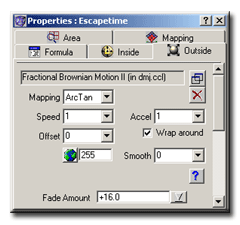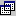Selecting a Coloring Formula: By pressing this button a modal dialog box will appear: It will display all coloring formulas currently known to ChaosPro. Select a formula and double click onto the formula name to assign it to the fractal. If you hold down the “SHIFT”-key on your keyboard, the current parameter values are preserved and the new formula will use that values.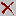Removing a Coloring Formula: If you press this button then the coloring formula currently assigned to the fractal will be removed. A default algorithm will be used instead.

As mentioned before, the coloring algorithm returns an index. ChaosPro now transforms this “index” value according to the settings specified in the coloring tab and then returns the color of the gradient at the resulting index.
The “transformations” done by ChaosPro are simply some density transformations, so that the color density is affected.

The most important setting is the “Mapping” function. The images below show the same image using the same gradient, but with different mapping functions: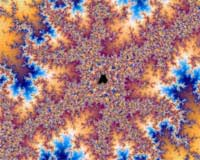Mapping=LinearMapping=Sqrt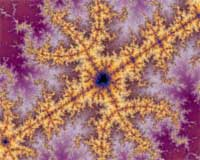Mapping=Arctan

As you can see, the mapping function has great influence on the color density.
The following options exist:

 None: Simply returns the solid color. The coloring algorithm has no influence Linear: Just returns the index value: The color density won’t be affected. Sqr: Returns the square of the index value. The heigher the index, the higher the color density. Sqrt: Returns the square root of the index value. The heigher the index, the lower the color density. Cube: Returns the square root of the index value. The heigher the index, the higher the color density. Cuberoot: Returns the square root of the index value. The heigher the index, the lower the color density. Exp: Returns exp(index). The heigher the index, the higher the color density. Log: Returns log(index). The heigher the index, the lower the color density. Sin: Returns the sine of the index. The color density changes in a periodic manner. Arctan: Returns the arcus tangent of the index value. The heigher the index, the lower the color density.

In addition to the mapping function the settings “Speed” and “Accel” exist:

• Speed multiplies the index value: So Speed>1 increases the color density, whereas Speed<1 decreases the color density.
• The “Accel” setting is not used by all mapping functions: Normally it lets you change the break-even between color densities, so it divides the resulting index value.

Some more options exist: They allow you to customize the use of colors even more:

• The “Offset” setting lets you specify an offset to use for the gradient.
• “Wrap around”: If checked, the gradient will be continuously repeated:
Normally “index value”=0 gets mapped to color 0, “index value”=1 gets mapped to last color of color map.
If “Wrap around” is not checked, then all (resulting) index values greater than 1 will be mapped to the last color of the gradient.
If “Wrap around” is checked, then the gradient will be repeated.
• The “Smooth” setting gives you control over the color interpolation: The color map has entries ranging from 0 to 249. The resulting index value can be any floating point number. Smooth=1 means, that the resulting index value will be rounded to a multiply of 1, i.e. to the next integer number. Then the index is used to extract the color.
Smooth=0.5 means, that the resulting index will be rounded to a multiply of 0.5, meaning that the final index used for color extraction from the color map can have values 0, 0.5, 1, 1.5, 2 … Smooth=0.1 means, that the resulting index will be rounded to a multiply of 0.1, meaning that the final index used for color extraction from the color map can have values 0, 0.1, 0.2, 0.3, 0.4 … Smooth=0 means, that the resulting index will not be rounded at all, it is already the final index used for extracting the color from the color map…